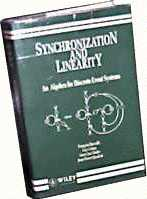Synchronization and Linearity : An Algebra for Discrete Event Systems F. BACCELLI, INRIA, Sophia Antipolis, France, now with ENS Ulm, Paris, France G. COHEN, Ecole des Mines de Paris, Fontainebleau, France, now with CERMICS-ENPC, Marne la Vallée, France G.J. OLSDER, Delft University of Technology, The Netherlands, J.-P. QUADRAT, INRIA, Rocquencourt, France.

NOW OUT OF PRINT: you can get an electronic version of this book here

This book proposes a unified mathematical treatment of a class of 'linear' discrete event systems, which contains important subclasses of Petri nets and queuing networks with synchronization constraints. The linearity has to be understood with respect to nonstandard algebraic structures, e.g. the 'max-plus algebra'. A calculus is developed based on such structures, which is followed by tools for computing the time behaviour to such systems. This algebraic vision lays the foundation of a bona fide 'discrete event system theory', which is shown to parallel the classical linear system theory in several ways.

This class of synchronized systems finds its main current industrial applications in the modeling, optimization and control of communications networks, computer architectures, manufacturing and transportation systems. The book should hence appeal to students and researchers in probability-statistics, applied mathematics, (industrial and electrical) engineering, operations research, as well as computer and management scientists.

CONTENTS

• Preface
• PART I: DISCRETE EVENT SYSTEMS AND PETRI NETS
• Introduction and Motivation
• Graph Theory and Petri Nets
• PART II: ALGEBRA
• Max-Plus Algebra
• Dioids
• PART III: DETERMINISTIC SYSTEM THEORY
• Two-dimensional Domain Description of Event Graphs
• Max-Plus Linear System Theory
• PART IV: STOCHASTIC SYSTEMS
• Ergodic Theory of Event Graphs
• Computational Issues in Stochastic Event
• Graphs
• PART V: POSTFACE
• Related Topics and Open Ends
• Bibliography
• Notation
• Index.
Wiley Series in Probability and Mathematical Statistics
ISBN 0 471 93609 X, 514 pp, August 1992

John Wiley & Sons Ltd,
Baffins Lane,
Chichester, West Sussex P019 IUD. UK
Telephone: (0243) 779777 Fax: (0243) 775878
REGISTERED NO: 641132 ENGLAND